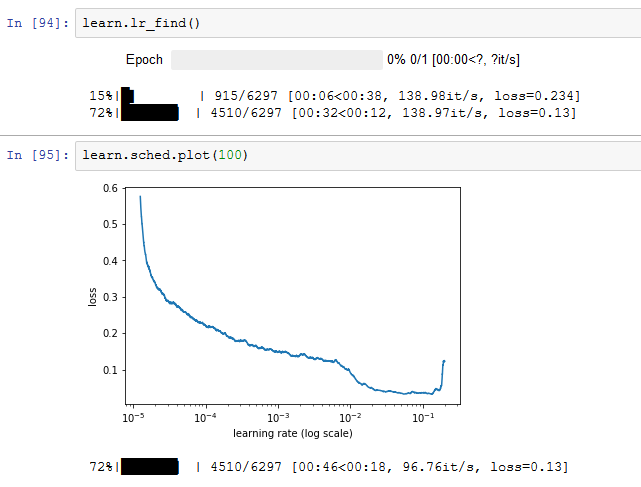# Setup of StructuredLearner and different results

I create two models with the same data and get different, but consistent results.
Model A is taken straight from lesson 3.
Model B is a variation that I can use to make predictions.

``````mdA = ColumnarModelData.from_data_frame(PATH, val_idx, df, yl.astype(np.float32), cat_flds=cat_vars, bs=128, test_df=df_test)
m = mdA.get_learner(emb_szs, len(df.columns)-len(cat_vars),
0.04, 1, [1000,500], [0.001,0.01], y_range=y_range)
``````

Notice that the configuration is the same.

``````mixedinputmodel = MixedInputModel(emb_szs, len(df.columns)-len(cat_vars),
0.04, 1, [1000,500], [0.001,0.01], y_range=y_range).cuda()
basicmodel = BasicModel(mixedinputmodel, 'mixedInputRegression')
mdB = ColumnarModelData.from_data_frame(PATH, val_idx, df, yl.astype(np.float32), cat_flds=cat_vars, bs=128, test_df=df_test)
learn = StructuredLearner(mdB, basicmodel)
``````

Here it gets interesting: m.model and learn.model are give the same result, but (m.model == learn.model) returns False.

``````m.model #as well as learn.model

>>>MixedInputModel(
(embs): ModuleList(
(0): Embedding(1116, 50)
(1): Embedding(8, 4)
(2): Embedding(4, 2)
(3): Embedding(13, 7)
(4): Embedding(32, 16)
(5): Embedding(3, 2)
(6): Embedding(26, 13)
(7): Embedding(27, 14)
(8): Embedding(5, 3)
(9): Embedding(4, 2)
(10): Embedding(4, 2)
(11): Embedding(24, 12)
(12): Embedding(9, 5)
(13): Embedding(13, 7)
(14): Embedding(53, 27)
(15): Embedding(22, 11)
(16): Embedding(1, 1)
(17): Embedding(1, 1)
(18): Embedding(1, 1)
(19): Embedding(1, 1)
(20): Embedding(1, 1)
(21): Embedding(1, 1)
)
(lins): ModuleList(
(0): Linear(in_features=201, out_features=1000, bias=True)
(1): Linear(in_features=1000, out_features=500, bias=True)
)
(bns): ModuleList(
(0): BatchNorm1d(1000, eps=1e-05, momentum=0.1, affine=True)
(1): BatchNorm1d(500, eps=1e-05, momentum=0.1, affine=True)
)
(outp): Linear(in_features=500, out_features=1, bias=True)
(emb_drop): Dropout(p=0.04)
(drops): ModuleList(
(0): Dropout(p=0.001)
(1): Dropout(p=0.01)
)
(bn): BatchNorm1d(18, eps=1e-05, momentum=0.1, affine=True)
)
``````

Then I find the learning rate:
The model A from @jeremy’s code always yields ~1e-4.

(Model B gives ~1e-2).My question is: lr_find() gives different results. What is the difference between these two approaches?
The reason why I bother with Model B is because I can do this:

``````my = learn.model
predict(my,e)
``````

Where e is a generator with data for prediction. (Final tensor ‘1110’ is a dummy y value - I had to add it or predict wouldn’t work. It doesn’t seem to do anything)

[

Columns 0 to 12
1 3 2 9 17 1 25 1 3 1 0 16 1

Columns 13 to 21
5 38 0 0 0 0 0 0 0
[torch.cuda.LongTensor of size 1x22 (GPU 0)],

Columns 0 to 9
-0.5358 1.2429 1.2198 1.0903 0.8733 0.4603 -0.7045 -0.7387 -0.3113 -2.1910

Columns 10 to 17
-0.7198 -0.8480 0.0000 0.0000 1.1137 -0.4899 -0.0509 -0.2961
[torch.cuda.FloatTensor of size 1x18 (GPU 0)],
1110
[torch.cuda.LongTensor of size 1 (GPU 0)]]

I have just realized that I can do this:
`predict(m.model, e)`
but I think the question is still valid.